Courses

# MCQ (Previous Year Questions) - Organic Chemistry (Level 2)

## 20 Questions MCQ Test Chemistry for JEE Advanced | MCQ (Previous Year Questions) - Organic Chemistry (Level 2)

Description
This mock test of MCQ (Previous Year Questions) - Organic Chemistry (Level 2) for JEE helps you for every JEE entrance exam. This contains 20 Multiple Choice Questions for JEE MCQ (Previous Year Questions) - Organic Chemistry (Level 2) (mcq) to study with solutions a complete question bank. The solved questions answers in this MCQ (Previous Year Questions) - Organic Chemistry (Level 2) quiz give you a good mix of easy questions and tough questions. JEE students definitely take this MCQ (Previous Year Questions) - Organic Chemistry (Level 2) exercise for a better result in the exam. You can find other MCQ (Previous Year Questions) - Organic Chemistry (Level 2) extra questions, long questions & short questions for JEE on EduRev as well by searching above.
QUESTION: 1

Solution:
QUESTION: 2

### Which of the following acids has the smallest dissociation constant ?                 [JEE-2002]

Solution:
The acidity increases with the increase in electronegativity of the halogen present.
Smallest dissociation constant means weakest acid, which is BrCH2 CH2OOH because here Br (less electronegative than F ) is two carbon atoms away from –COOH.
QUESTION: 3

### Which of the following represent the given mode of hybridisation sp2 – sp2 – sp – sp from left to right?            [JEE-2003]

Solution:
QUESTION: 4

Maximum dipolemoment will be of            [JEE-2003]

Solution: -This is due to in CH3Cl chlorine is EWG and it is in one direction and no other group present for cancelling/decreasing its dipole moment. 2- In CH2Cl2 dipole moment of H-H atoms and Cl-Cl atoms do not cancel each other because angle are not 180 deg so they are not linear. 3- In CCl4 dipole moment cancel and become zero because angle between trans position of Cl atom is 180deg.
QUESTION: 5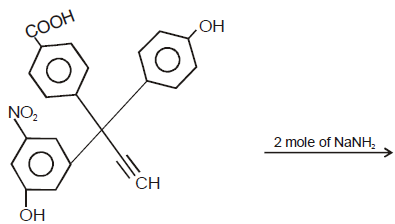Product (A). The product A will be                      [JEE-2003]

Solution:
QUESTION: 6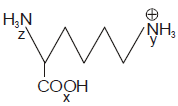[JEE-2004]

Correct order of acidic strength is

Solution:
QUESTION: 7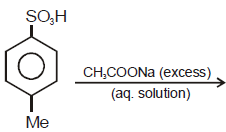[JEE-2005]

Solution: The H atom of SO3H will get attached to CH3COONA and will become CH3COOH and this will get attached to benzene ring and the SO3Na will also formed
QUESTION: 8

For 1-methoxy-1,3-butadiene, which of the following resonating structure is the least stable ?       [JEE-2005]

Solution:

There is no conjugation stability, two double bonds are together and -ve charge & lone pair (on oxygen) are also together in option C

Also carbon having + charge form more than 4 bonds, thus breaking the rule of tetra-valancy.

Hence C

QUESTION: 9

When benzene sulfonic acid and p-nitrophenol are treated with NaHCO3, the gases released respectively are [JEE-2006]

Solution:
Co2.
because they are acids and any acid releases co2 from bicarbonates.
QUESTION: 10

(I) 1, 2 - dihydroxy beznene
(II) 1, 3 - dihydroxy benzene
(III) 1, 4 - dihydroxy benzene
(IV) Hydroxy benzene
The increasing order of boiling points of above mentioned alcohols is               [JEE-2006]

Solution: (c) Among the given compounds, hydroxybenzene (IV) has least molar mass and therefore posses least boiling point. Among the three isomeric dihydroxybenzenes, 1,2- dihydroxybenzene (I) forms intramolecural H-bonding with the result it will not form intermolecular H-bonding leading to lowest boiling point. On the other hand 1,3-dihydroxybenzene (II) and 1,4-dihyroxybenzene (III)do not undergo chelation, hence they will involve extensive intermolecular H-bonding leading to higher boiling point. Further intermolecular hydrogen bonding is stronger in the p-isomer than the m-isomer hence former has highest b.p. Thus the deacreasing order of boiling points is III > II > I >IV.
QUESTION: 11

Among the following, the least stable resonance structur is                     [JEE-2007]

Solution: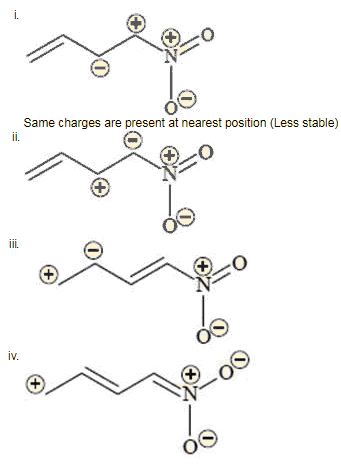Hence (A) is correct
QUESTION: 12

Statement-1: p-Hydroxybenzoic acid has a lower boiling point then o-hydroxybenzoic acid.

Statement-2: o-Hydroxybenzoic acid has a intramoleculer hydrogen bonding.             [JEE-2007]

Solution: As the number of hydrogen bonding increases the boiling point increases. In p hydroxy benzoic acid more hydrogen bonds can be formed as both carboxylic group and hydroxyl group forms intermolecular hydrogen bonding but in o hydroxy benzoic acid intramolecular hydrogen bonding thus number of hydrogen bonding decreases thus boiling point decreases that is its boiling point is lower than p hydroxy benzoic acid . Thus statement 1 is false but statement 2 is true.
QUESTION: 13

Hyperconjugation involves overlap of the following orbitals                [JEE-2008]

Solution:
QUESTION: 14

The correct stability order for the following species is                    [JEE-2008]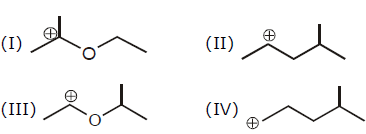Solution:
QUESTION: 15

The correct acidity order of the following is                      [JEE-2009]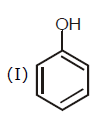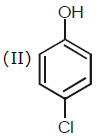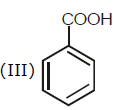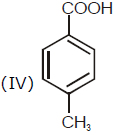Solution:
QUESTION: 16

The correct stability order of the following resonance structures is                [JEE-2009]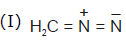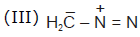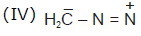Solution:
QUESTION: 17

In the following carbocation; H/CH3 that is most likely to migrate to the positively charged carbon is [JEE-2009]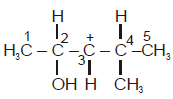Solution:
QUESTION: 18

Among the following compounds, the most acidic is:                   [JEE-2011]

Solution: Ortho-isomer, a strong hydrogen bridge can be formed between the phenolic -OH and the the carboxylate anion. This will tend to stabilize the conjugated carboxylate base, and hence will increase acidity.

this is the order of acidic

ortho hydroxy B.acid > meta hydroxy B.acid > benzoic acid > para hydroxy B. acids.
QUESTION: 19

In Allen (C3H4), the type (s) of hybridisation of the carbon atoms is (are)              [JEE-2012]

Solution: In allene we can tell hybridization separately for different c-atom not of the the molecule .The two c atom H2-c=c=c-H2 at terminal have sp2 while the middle have sp .
QUESTION: 20

Which of the following molecules in pure from is (are) unstable at room temperature                  [JEE-2012]

Solution: